# Logical Reasoning and Analytical Ability (Test 4)

## Upsc Civil Services Entrance Exams : General Studies Test

| Home | | Upsc Civil Services Entrance Exams | | General Studies Test | | Logical Reasoning and Analytical Ability |Logical Reasoning and Analytical Ability
| Logical Reasoning | | Analytical Reasoning |
Q.1
Find the number of triangles in the given figure.A. 12
B. 18
C. 22
D. 26
Explaination / Solution:

The figure may be labelled as shown.The simplest triangles are AHB, GHI, BJC, GFE, GIE, IJE, CEJ and CDE i.e. 8 in number.

The triangles composed of two components each are HEG, BEC, HBE, JGE and ICE i.e. 5 in number.

The triangles composed of three components each are FHE, GCE and BED i.e. 3 in number.

There is only one triangle i.e. AGC composed of four components.

There is only one triangle i.e. AFD composed of nine components.

Thus, there are 8 + 5 + 3 + 1 + 1 = 18 triangles in the given figure.

Workspace
Report
Q.2
Find the number of triangles in the given figure.A. 12
B. 13
C. 14
D. 15
Explaination / Solution:

The figure may be labelled as shown.The simplest triangles are ABF, BIC, CIH, GIH, FGE and AFE i.e. 6 in number.

The triangles composed of two components each are ABE, AGE, BHF, BCH, CGH and BIE i.e. 6 in number.

The triangles composed of three components each are ABH, BCE and CDE i.e. 3 in number.

Hence, the total number of triangles in the figure = 6 + 6 + 3 = 15.

Workspace
Report
Q.3
Find the minimum number of straight lines required to make the given figure.A. 9
B. 11
C. 15
D. 16
Explaination / Solution:

The figure may be labelled as shown.The horizontal lines are DE, FH, IL and BC i.e. 4 in number.

The slanting lines are AC, DO, FN, IM, AB, EM and HN i.e. 7 in number.

Thus, there are 4 + 7 = 11 straight lines in the figure.

Workspace
Report
Q.4

Find the number of triangles in the given figure.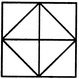A. 8
B. 10
C. 12
D. 14
Explaination / Solution:

The figure may be labelled as shown.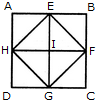The simplest triangles are AEH, EHI, EBF, EFI, FGC, IFG, DGH and HIG i.e. 8 in number.

The triangles composed of two components each are HEF, EFG, HFG and EFG i.e. 4 in number.

Thus, there are 8 + 4 = 12 triangles in the figure.

Workspace
Report
Q.5

Find the minimum number of straight lines required to make the given figure.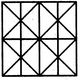A. 11
B. 14
C. 16
D. 17
Explaination / Solution:

The figure may be labelled as shown.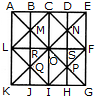The horizontal lines are AK, BJ, CI, DH and EG i.e. 5 in number.

The vertical lines are AE, LF and KG i.e. 3 in number.

The slanting lines are LC, CF, FI, LI, EK and AG i.e. 6 in number.

Thus, there are 5 + 3 + 6 = 14 straight lines in the figure.

Workspace
Report
Q.6

Find the number of triangles in the given figure.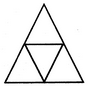A. 4
B. 5
C. 6
D. 7
Explaination / Solution:

The figure may be labelled as shown.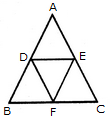The simplest triangles are ADE, BDF, DEF and EFC i.e. 4 in number.

There is only one triangle ABC composed of four components.

Thus, there are 4+1 = 5 triangles in the given figure.

Workspace
Report
Q.7

Count the number of squares in the given figure.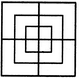A. 8
B. 12
C. 15
D. 18
Explaination / Solution:

The figure may be labelled as shown.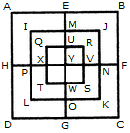The simplest squares are QUYX, URVY, YVSW and XYWT i.e. 4 in number.

The squares composed of two components each are IMYP, MJNY, YNKO and PYOL i.e. 4 in number.

The squares composed of three components each are AEYH, EBFY, YFCG and HYGD i.e. 4 in number.

There is only one square i.e. QRST composed of four components.

There is only one square i.e. IJKL composed of eight components.

There is only one square i.e. ABCD composed of twelve components.

Total number of squares in the given figure = 4 + 4 + 4+1 + 1 + 1 = 15.

Workspace
Report
Q.8

Count the number of triangles and squares in the given figure.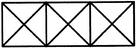A. 28 triangles, 3 squares
B. 24 triangles, 5 squares
C. 28 triangles, 5 squares
D. 24 triangles, 3 squares
Explaination / Solution:

The figure may be labelled as shown.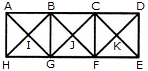Triangles :

The, simplest triangles are ABI, BGI, GHI, HAI, BCJ, CFJ, FGJ, GBJ, CDK, DEK, EFK and FCK i.e. 12 in number.

The triangles composed of two components each are ABG, BGH, GHA, HAB, BCF, CFG, FGB, GBC, CDE, DEF, EFC and FGD i.e. 12 in number.

The triangles composed of four components each are AGC, BFD, HBF and GCE i.e.4 in number.

Thus, there are 12 + 12 + 4 = 28 triangles in the given figure.

Squares :

The squares composed of two components each are BJGI and CKFJ i.e. 2 in number.

The squares composed of four components each are ABGH, BCFG and CDEF i.e. 3 in number.

Total number of squares in the figure = 2 + 3 = 5.

Workspace
Report
Q.9

How many triangles and parallelograms are there in the following figure?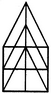A. 21, 17
B. 19, 13
C. 21, 15
D. 19, 17
Explaination / Solution:

The figure may be labelled as shown.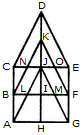Triangles:

The simplest triangles are KJN, KJO, CNB, OEF, JIL, JIM, BLA and MFG i.e. 8 in number.

The triangles composed of two components each are CDJ, EDJ, NKO, JLM, JAH and JGH i.e. 6 in number.

The triangles composed of three components each are BKI, FKI, CJA and EJG i.e. 4 in number.

The triangles composed of four components each are CDE and AJG i.e. 2 in number.

The only triangle composed of six components is BKF.

Thus, there are 8 + 6 + 4 + 2 + 1 = 21 triangles in the given figure.

Parallelograms :

The simplest parallelograms are NJLB and JOFM i.e. 2 in number.

The parallelograms composed of two components each are CDKB, DEFK, BIHA and IFGH i.e.4 in number.

The parallelograms composed of three components each are BKJA, KFGJ, CJIB and JEFI i.e.4 in number.

There is only one parallelogram i.e. BFGA composed of four components.

The parallelograms composed of five components each are CDJA, DEGJ, CJHA and JEGH i.e.4 in number.

The only parallelogram composed of six components is CEFB.

The only parallelogram composed of ten components is CEGA.

Thus, there are 2 + 4 + 4 + 1 + 4+ 1 + 1 = 17 parallelograms in the given figure.

(Here note that the squares and rectangles are also counted amongst the parallelograms).

Workspace
Report
Q.10

Count the number of triangles and squares in the given figure.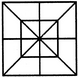A. 28 triangles, 10 squares
B. 28 triangles, 8 squares
C. 32 triangles, 10 squares
D. 32 triangles, 8 squares
Explaination / Solution:

The figure may be labelled as shown.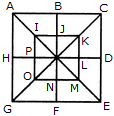Triangles :

The simplest triangles are IJQ, JKQ, KLQ, LMQ, MNQ, NOQ, OPQ and PIQ i.e. 8 in number. The triangles composed of two components each are ABQ, BCQ, CDQ, DEQ, EFQ, FGQ, GHQ, HAQ, IKQ, KMQ, MOQ and OIQ i.e. 12 in number.

The triangles composed of four components each are ACQ, CEQ, EGQ, GAQ, IKM, KMO, MOI and OIK i.e. 8 in number.

The triangles composed of eight components each are ACE, CEG, EGA and GAC i.e. 4 in number.

Total number of triangles in the figure = 8 + 12 + 8 + 4 = 32.

Squares :

The squares composed of two components each are IJQP, JKLQ, QLMN and PQNO i.e. 4 in number.

The squares composed of four components each are ABQH, BCDQ, QDEF and HQFG i.e. 4 in number.

There is only one square i.e. IKMO composed of eight components.

There is only one square i.e. ACEG composed of sixteen components.

Thus, there are 4 + 4 + 1 + 1= 10 squares in the given figure.

Workspace
Report